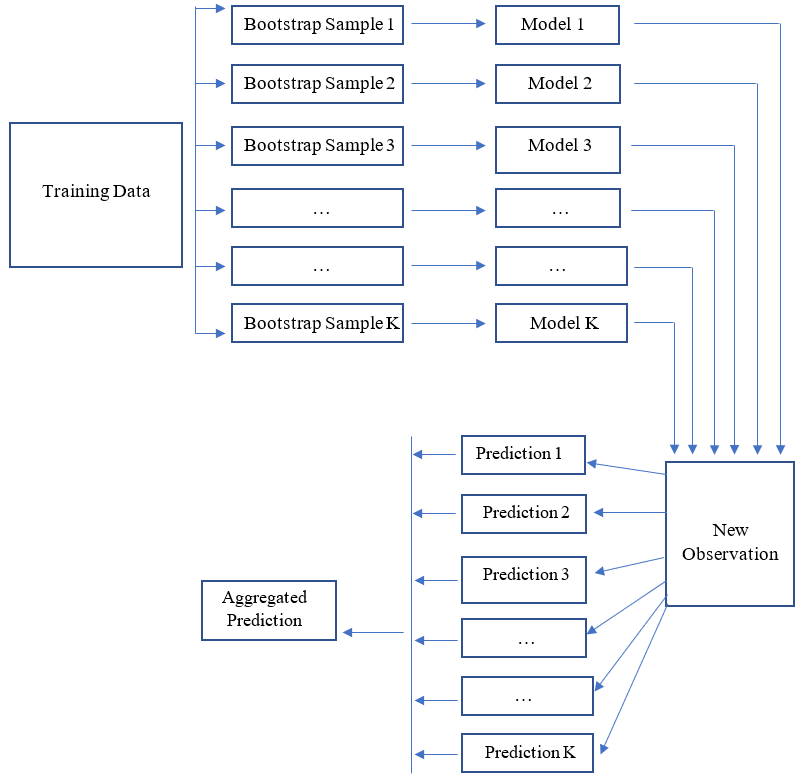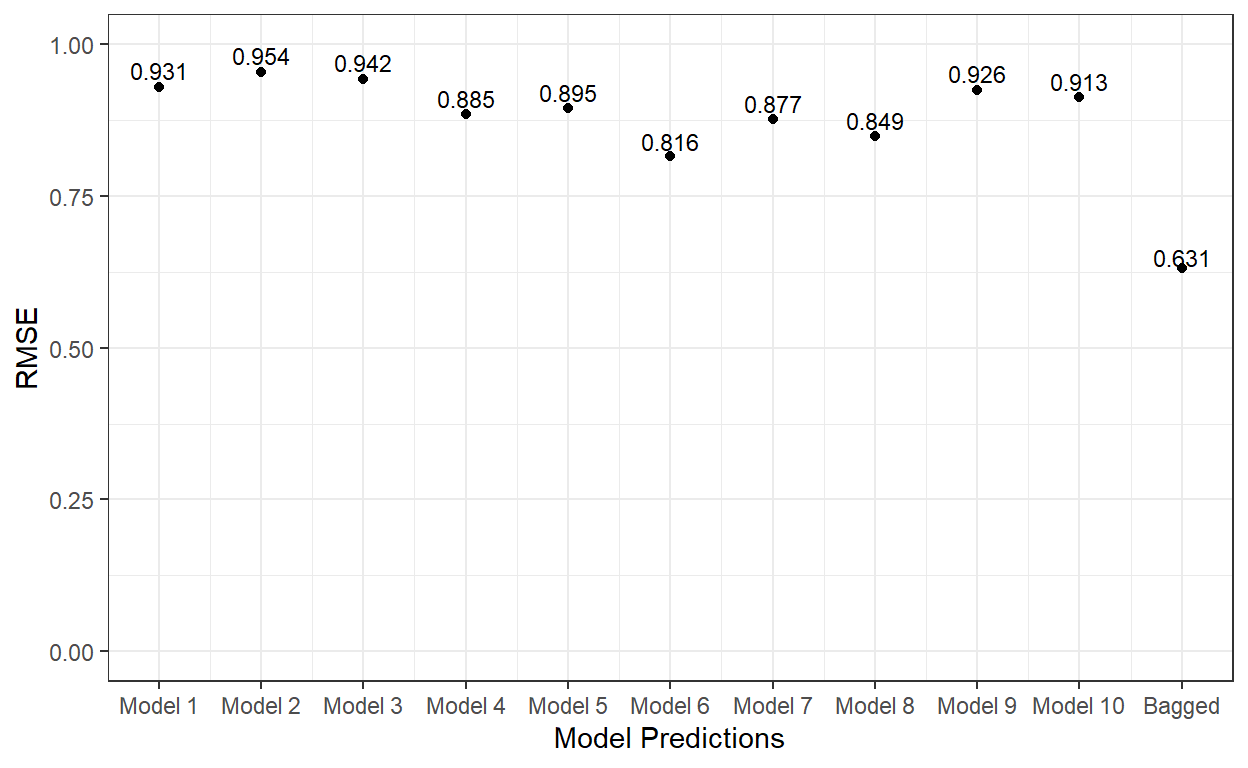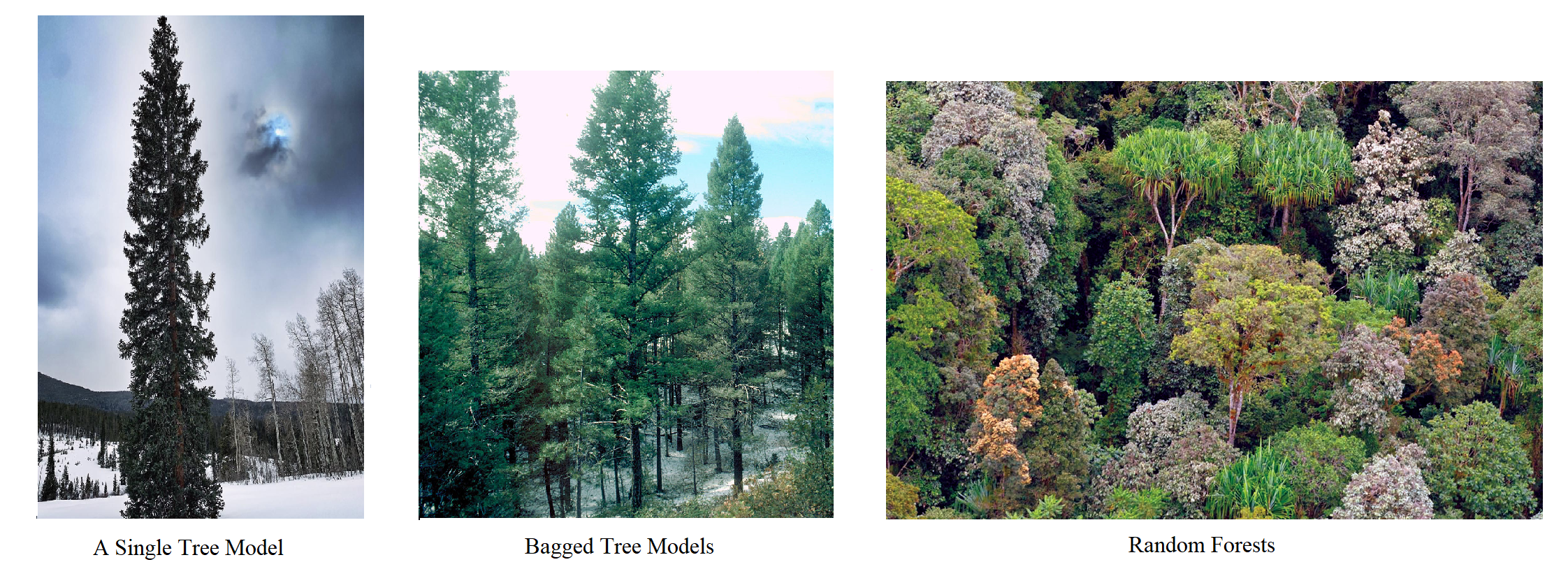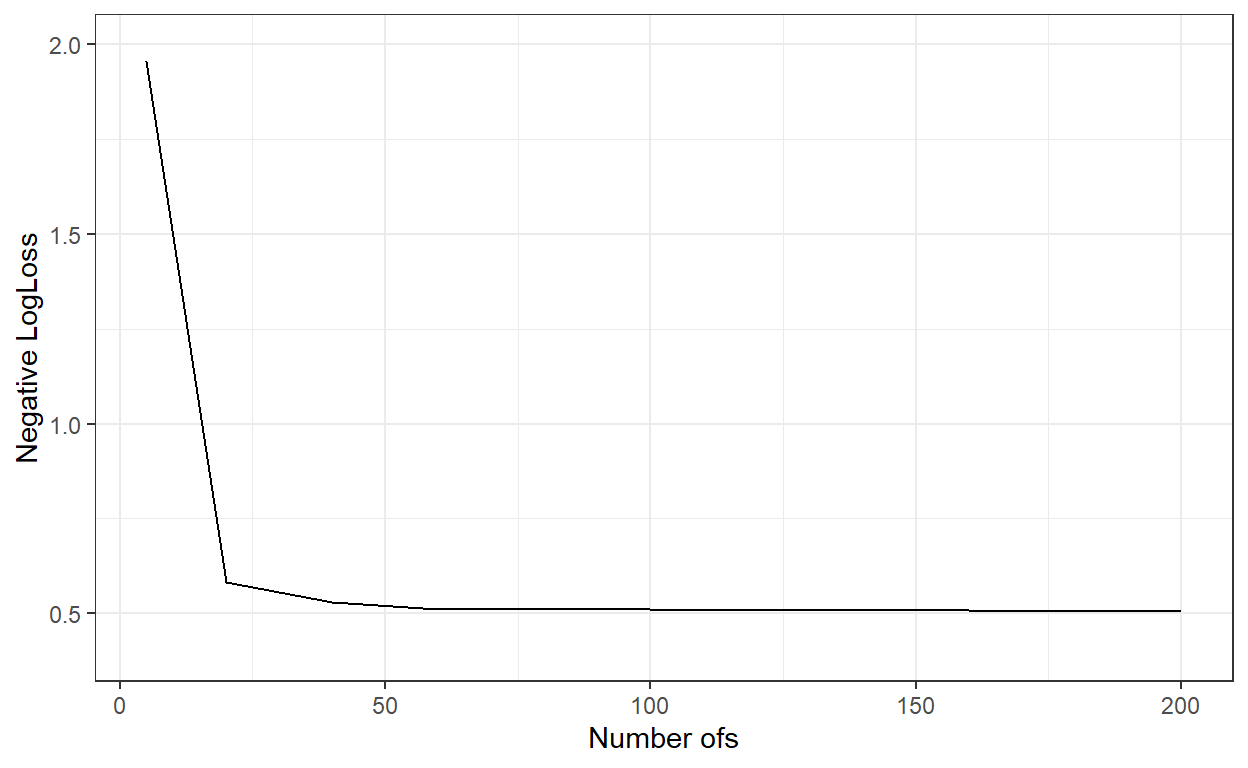# Bagged Trees and Random Forests

Cengiz Zopluoglu (University of Oregon)
11/23/2022

[Updated: Sun, Nov 27, 2022 - 14:10:08 ]

# 1. The Concept of Bootstrap Aggregation (BAGGING)

The concept of bagging is based on the idea that predictions from an ensemble of models are better than any single model predictions. Suppose we randomly draw multiple samples from a population and then develop a prediction model for an outcome using each sample. The aggregated predictions from these multiple models would perform better due to the reduced model variance (aggregation would reduce noise due to sampling).

Due to the lack of access to the population (even if we assume there is a well-defined population), we can mimic the sampling from a population by replacing it with bootstrapping. A Bootstrap sample is a random sample with replacement from the sample data.

Suppose there is sample data with ten observations and three predictors. Below are five bootstrap samples from this sample data.

``````d <- data.frame(x1 =c(20,25,30,42,10,60,65,55,80,90),
x2 =c(10,15,12,20,45,75,70,80,85,90),
x3 =c(25,30,35,20,40,80,85,90,92,95),
label= c('A','B','C','D','E','F','G','H','I','J'))

d``````
``````   x1 x2 x3 label
1  20 10 25     A
2  25 15 30     B
3  30 12 35     C
4  42 20 20     D
5  10 45 40     E
6  60 75 80     F
7  65 70 85     G
8  55 80 90     H
9  80 85 92     I
10 90 90 95     J``````
``````set.seed(11232022)

# Bootstrap sample 1

d[sample(1:10,replace = TRUE),]``````
``````     x1 x2 x3 label
2    25 15 30     B
2.1  25 15 30     B
6    60 75 80     F
1    20 10 25     A
8    55 80 90     H
10   90 90 95     J
10.1 90 90 95     J
8.1  55 80 90     H
10.2 90 90 95     J
3    30 12 35     C``````
``````# Bootstrap sample 2

d[sample(1:10,replace = TRUE),]``````
``````     x1 x2 x3 label
4    42 20 20     D
9    80 85 92     I
9.1  80 85 92     I
10   90 90 95     J
6    60 75 80     F
10.1 90 90 95     J
3    30 12 35     C
2    25 15 30     B
9.2  80 85 92     I
4.1  42 20 20     D``````
``````# Bootstrap sample 3

d[sample(1:10,replace = TRUE),]``````
``````    x1 x2 x3 label
9   80 85 92     I
3   30 12 35     C
6   60 75 80     F
3.1 30 12 35     C
4   42 20 20     D
5   10 45 40     E
4.1 42 20 20     D
8   55 80 90     H
10  90 90 95     J
3.2 30 12 35     C``````
``````# Bootstrap sample 4

d[sample(1:10,replace = TRUE),]``````
``````     x1 x2 x3 label
8    55 80 90     H
7    65 70 85     G
3    30 12 35     C
1    20 10 25     A
10   90 90 95     J
2    25 15 30     B
9    80 85 92     I
10.1 90 90 95     J
6    60 75 80     F
10.2 90 90 95     J``````
``````# Bootstrap sample 5

d[sample(1:10,replace = TRUE),]``````
``````    x1 x2 x3 label
3   30 12 35     C
10  90 90 95     J
5   10 45 40     E
8   55 80 90     H
6   60 75 80     F
3.1 30 12 35     C
1   20 10 25     A
4   42 20 20     D
9   80 85 92     I
8.1 55 80 90     H``````

The process of bagging is building separate models for each bootstrap sample and then applying all these models to a new observation for predicting the outcome. Finally, these predictions are aggregated in some form (e.g., taking the average) to obtain a final prediction for the new observation. The idea of bagging can technically be applied to any prediction model (e.g., CNN’s, regression models). During the model process from each bootstrap sample, no regularization was applied, and models were developed to their full complexity. So, we obtain so many unbiased models. While each model has a significant sample variance, we hope to reduce this sampling variance by aggregating the predictions from all these models at the end.## 1.1. BAGGING: Do It Yourself with the `rpart` package

In this section, we will apply the bagging idea to decision trees to predict the readability scores. First, we import and prepare data for modeling. Then, we split the data into training and test pieces.

``````# Import the dataset

# Write the recipe

require(recipes)

roles = c(rep('predictor',768),'outcome')) %>%
step_zv(all_numeric()) %>%
step_nzv(all_numeric()) %>%
step_normalize(all_numeric_predictors())

# Train/Test Split

set.seed(10152021)  # for reproducibility

The code below will take a

1. bootstrap sample from training data,
1. develop a full tree model with no pruning, and
1. save the model object as an element of a list.

We will repeat this process ten times.

``````require(caret)

bag.models <- vector('list',10)

for(i in 1:10){

# Bootstrap sample

# Train the tree model with no pruning and no cross validation

grid <- data.frame(cp=0)
cv <- trainControl(method = "none")

data      = temp,
method    = 'rpart',
tuneGrid  = grid,
trControl = cv,
control   = list(minsplit=20,
minbucket = 2,
maxdepth = 60))

}``````

Now, we will use each of these models to predict the readability score for the test data. We will also average these predictions. Then, we will save the predictions in a matrix form to compare.

``````preds <- data.frame(obs = read_te[,c('target')])

preds\$average <- rowMeans(preds[,2:11])

``````     obs model1 model2 model3 model4 model5 model6 model7 model8
1  0.246 -0.827  0.355  0.619 -0.925 -0.956  0.260 -0.095 -0.224
2 -0.188 -1.399 -0.963 -0.458  0.635 -0.415 -0.684 -0.101 -0.963
3 -0.135 -0.235  1.149  0.827  0.663  0.015  0.865  0.693 -0.013
4  0.395  0.690  0.355  1.361  0.025 -0.271  0.119 -0.406 -0.044
5 -0.371 -1.232 -0.901 -1.968 -1.147 -1.420 -1.393 -1.104  0.114
6 -1.156 -0.173 -0.531 -0.694 -1.390 -0.508 -0.690 -0.769  0.360
model9 model10 average
1 -0.610   0.433  -0.197
2 -0.610   0.259  -0.470
3  0.242  -0.253   0.395
4  0.005   0.739   0.257
5 -1.024  -1.125  -1.120
6 -0.252  -0.894  -0.554``````

Now, let’s compute the RMSE for each model’s predicted scores and the RMSE for the average of predicted scores from all ten tree models.

``````p1 <- sqrt(mean((preds\$obs - preds\$model1)^2))
p2 <- sqrt(mean((preds\$obs - preds\$model2)^2))
p3 <- sqrt(mean((preds\$obs - preds\$model3)^2))
p4 <- sqrt(mean((preds\$obs - preds\$model4)^2))
p5 <- sqrt(mean((preds\$obs - preds\$model5)^2))
p6 <- sqrt(mean((preds\$obs - preds\$model6)^2))
p7 <- sqrt(mean((preds\$obs - preds\$model7)^2))
p8 <- sqrt(mean((preds\$obs - preds\$model8)^2))
p9 <- sqrt(mean((preds\$obs - preds\$model9)^2))
p10 <- sqrt(mean((preds\$obs - preds\$model10)^2))

p.ave <- sqrt(mean((preds\$obs - preds\$average)^2))

ggplot()+
geom_point(aes(x = 1:11,y=c(p1,p2,p3,p4,p5,p6,p7,p8,p9,p10,p.ave)))+
xlab('Model Predictions') +
ylab('RMSE') +
ylim(0,1) +
scale_x_continuous(breaks = 1:11,
labels=c('Model 1','Model 2', 'Model 3', 'Model 4',
'Model 5','Model 6', 'Model 7', 'Model 8',
'Model 9','Model 10','Bagged'))+
theme_bw()+
annotate('text',
x = 1:11,
y=c(p1,p2,p3,p4,p5,p6,p7,p8,p9,p10,p.ave)*1.03,
label = round(c(p1,p2,p3,p4,p5,p6,p7,p8,p9,p10,p.ave),3),
cex=3)``````As it is evident, the bagging of 10 different tree models significantly improved the predictions on the test dataset.

## 1.2. BAGGING with the `ranger` and `caret::train()` packages

Instead of writing your code to implement the idea of bagging for decision trees, we can use the `ranger` method via `caret::train()`.

``````require(ranger)

getModelInfo()\$ranger\$parameters``````
``````      parameter     class                         label
1          mtry   numeric #Randomly Selected Predictors
2     splitrule character                Splitting Rule
3 min.node.size   numeric             Minimal Node Size``````

The `caret::train()` allows us manipulate three parameters while using the `ranger` method:

• splitrule: set this to ‘variance’ for regression problems with continuous outcome. Other alternatives are `extratrees`, `maxstat`, and `beta`.

• min.node.size.: this is identical to `minbucket` argument in the `rpart` method and indicates the minimum number of observations for each node.

• mtry: this is the most critical parameter for this method and indicates the number of predictors to consider for developing tree models. For bagged decision trees, you can set this to the number of all predictors in your model

``````# Cross validation settings

# Create 10 folds with equal size

# Create the list for each fold

my.indices <- vector('list',10)
for(i in 1:10){
my.indices[[i]] <- which(folds!=i)
}

cv <- trainControl(method = "cv",
index  = my.indices)

# Grid, running with all predictors in the data (768)

grid <- expand.grid(mtry = 768,splitrule='variance',min.node.size=2)
grid``````
``````  mtry splitrule min.node.size
1  768  variance             2``````
``````# Bagging with 10 tree models

method    = 'ranger',
trControl = cv,
tuneGrid  = grid,
num.trees = 10,
max.depth = 60)``````

Let’s check the cross-validated performance metrics.

``bagged.trees\$results``
``````  mtry splitrule min.node.size      RMSE  Rsquared      MAE
1  768  variance             2 0.6804536 0.5683141 0.543069
RMSESD RsquaredSD      MAESD
1 0.02011178 0.02586802 0.01373682``````

The performance is very similar to what we got in our DIY demonstration.

A couple of things to note:

• When you set `max.depth=` argument within the `caret::train` function, it passes this to the `ranger` function. Try to set this number to as large as possible, so you develop each tree to its full capacity.

• The penalty term is technically zero (`cp` parameter in the `rpart` function) while building each tree model. In Bagging, we deal with the model variance differently. Instead of applying a penalty term, we ensemble many unpenalized tree models to reduce the model variance.

• The number of trees (bootstrap samples) is a hyperparameter to tune. Conceptually, the model performance will improve as you increase the number of tree models used in Bagging; however, the performance will stabilize at some point. It is a tuning process to find the minimum number of tree models in Bagging to obtain the maximal model performance.

## 1.3. Tuning the Number of Tree Models in Bagging

Unfortunately, `caret::train` does not let us define the `num.trees` argument as a hyperparameter in the grid search. So, the only way to search for the optimal number of trees is to use the `ranger` method via `caret::train` function and iterate over a set of values for the `num.trees` argument. Then, compare the model performance and pick the optimal number of tree models.

The code below implements this idea and saves the results from each iteration in a list object.

``````# Run the bagged trees by iterating over num.trees using the
# values 5, 20, 40, 60,  ..., 200

nbags <- c(5,seq(from = 20,to = 200, by = 20))

bags <- vector('list',length(nbags))

for(i in 1:length(nbags)){

method    = 'ranger',
trControl = cv,
tuneGrid  = grid,
num.trees = nbags[i],
max.depth = 60)

print(i)

}

# This can take a few hours to run.``````

Let’s check the cross-validated RMSE for the bagged tree models with different number of trees.

``````rmses <- c()

for(i in 1:length(nbags)){

rmses[i] = bags[[i]]\$results\$RMSE

}

ggplot()+
geom_line(aes(x=nbags,y=rmses))+
xlab('Number of Trees')+
ylab('RMSE')+
ylim(c(0.6,0.75))+
theme_bw()````````nbags[which.min(rmses)]``
`` 180``

It indicates that the RMSE stabilizes after roughly 60 tree models. We can see that a bagged tree model with 180 trees gave the best result. Let’s see how well this model performs on the test data.

``````# Predictions from a Bagged tree model with 180 trees

# MAE

`` 0.4776075``
``````# RMSE

`` 0.6003086``
``````# R-square

`` 0.6646118``

Now, we can add this to our comparison list to remember how well this performs compared to other methods.

R-square MAE RMSE
Linear Regression 0.658 0.499 0.620
Ridge Regression 0.727 0.432 0.536
Lasso Regression 0.721 0.433 0.542
Elastic Net 0.726 0.433 0.539
KNN 0.611 0.519 0.648
Decision Tree 0.499 0.574 0.724
Bagged Trees 0.664 0.478 0.600

# 2. Random Forests

Random Forests is an idea very similar to Bagging with an extra feature. In Random Forests, while we take a bootstrap sample of observations (a random sample of rows in training data with replacement), we also take a random sample of columns for each split while developing a tree model. It allows us to develop tree models more independently of each other.

When specific important predictors are related to the outcome, the tree models developed using all predictors will be very similar, particularly at the top nodes, although we take bootstrap samples. These trees will be correlated to each other, which may reduce the efficiency of BAGGING in reducing the variance. We can diversify the tree models by randomly sampling a certain number of predictors while developing each tree. It turns out that a diverse group of tree models does much better in predicting the outcome than a group of tree models similar to each other.We can use the same `ranger` package to fit the random forests models by only changing the `mtry` argument in our grid. Below, we will fit a random forests model with ten trees by randomly sampling from rows for each tree. In addition, when we develop each tree model, we will also randomly sample 300 predictors. I set `mtry=300` in the `grid` object, indicating that it will randomly sample 300 predictors to consider for each split when developing each tree.

``````# Grid, randomly sample 300 predictors

grid <- expand.grid(mtry = 300,splitrule='variance',min.node.size=2)
grid``````
``````  mtry splitrule min.node.size
1  300  variance             2``````
``````# Random Forest with 10 tree models

method    = 'ranger',
trControl = cv,
tuneGrid  = grid,
num.trees = 10,
max.depth = 60)

rforest\$times``````
``````\$everything
user  system elapsed
177.76    1.08  133.97

\$final
user  system elapsed
15.87    0.05   11.38

\$prediction
 NA NA NA``````

Let’s check the cross-validated performance metrics.

``rforest\$results``
``````  mtry splitrule min.node.size      RMSE  Rsquared       MAE
1  300  variance             2 0.6829234 0.5674509 0.5430574
RMSESD RsquaredSD      MAESD
1 0.01628784 0.02833995 0.01213438``````

For random forests, there are two hyperparameters to tune:

• `mtry`, the number of predictors to choose for each split during the tree model development

• `num.trees`, the number of trees.

As mentioned before, unfortunately, the `caret::train` only allows `mtry` in the grid search. For the number of trees, one should embed it in a `for` loop to iterate over a set of values. The code below hypothetically implements this idea by trying ten different `mtry` values (100,150,200,250,300,350,400,450,500,550) and saves the results from each iteration in a list object. However, I haven’t run it, which may take a long time.

``````# Grid Settings

grid <- expand.grid(mtry = c(100,150,200,250,300,350,400,450,500,550),
splitrule='variance',
min.node.size=2)

# Run the bagged trees by iterating over num.trees values from 1 to 200

bags <- vector('list',200)

for(i in 1:200){

method    = 'ranger',
trControl = cv,
tuneGrid  = grid,
num.trees = i,
max.depth = 60,)

}``````

Instead, I run this by fixing `mtry=300` and then iterating over the number of trees for values of 5, 20, 40, 60, 80, …, 200 (as we did for bagged trees).

``````rmses <- c()

for(i in 1:length(nbags)){

rmses[i] = bags[[i]]\$results\$RMSE

}

ggplot()+
geom_line(aes(x=nbags,y=rmses))+
xlab('Number of Trees')+
ylab('RMSE')+
ylim(c(0.6,0.75))+
theme_bw()````````nbags[which.min(rmses)]``
`` 160``

RMSE similarly stabilized after roughly 60 trees. Let’s see how well the model with 200 trees perform.

``````# Predictions from a Random Forest model with 160 trees

# MAE

`` 0.4756419``
``````# RMSE

`` 0.6003527``
``````# R-square

`` 0.6690829``

Below is our comparison table with Random Forests added. As you see, there is a slight improvement over Bagged Trees, and we can improve this a little more by trying different values of `mtry` and finding an optimal number.

R-square MAE RMSE
Linear Regression 0.658 0.499 0.620
Ridge Regression 0.727 0.432 0.536
Lasso Regression 0.721 0.433 0.542
Elastic Net 0.726 0.433 0.539
KNN 0.611 0.519 0.648
Decision Tree 0.499 0.574 0.724
Bagged Trees 0.664 0.478 0.600
Random Forests 0.669 0.476 0.600

# 3. Predicting Recidivism using Bagges Trees and Random Forests

In this section, I provide the R code to predict recidivism using Bagged Trees and Random Forests.

Import the recidivism dataset and pre-process the variables

``````# Import data

# Write the recipe

# List of variable types

outcome <- c('Recidivism_Arrest_Year2')

id      <- c('ID')

categorical <- c('Residence_PUMA',
'Prison_Offense',
'Age_at_Release',
'Supervision_Level_First',
'Education_Level',
'Prison_Years',
'Gender',
'Race',
'Gang_Affiliated',
'Prior_Arrest_Episodes_DVCharges',
'Prior_Arrest_Episodes_GunCharges',
'Prior_Conviction_Episodes_Viol',
'Prior_Conviction_Episodes_PPViolationCharges',
'Prior_Conviction_Episodes_DomesticViolenceCharges',
'Prior_Conviction_Episodes_GunCharges',
'Prior_Revocations_Parole',
'Prior_Revocations_Probation',
'Condition_MH_SA',
'Condition_Cog_Ed',
'Condition_Other',
'Violations_ElectronicMonitoring',
'Violations_Instruction',
'Violations_FailToReport',
'Violations_MoveWithoutPermission',
'Employment_Exempt')

numeric   <- c('Supervision_Risk_Score_First',
'Dependents',
'Prior_Arrest_Episodes_Felony',
'Prior_Arrest_Episodes_Misd',
'Prior_Arrest_Episodes_Violent',
'Prior_Arrest_Episodes_Property',
'Prior_Arrest_Episodes_Drug',
'Prior_Arrest_Episodes_PPViolationCharges',
'Prior_Conviction_Episodes_Felony',
'Prior_Conviction_Episodes_Misd',
'Prior_Conviction_Episodes_Prop',
'Prior_Conviction_Episodes_Drug',
'Delinquency_Reports',
'Program_Attendances',
'Program_UnexcusedAbsences',
'Residence_Changes',
'Avg_Days_per_DrugTest',
'Jobs_Per_Year')

props      <- c('DrugTests_THC_Positive',
'DrugTests_Cocaine_Positive',
'DrugTests_Meth_Positive',
'DrugTests_Other_Positive',
'Percent_Days_Employed')

# Convert all nominal, ordinal, and binary variables to factors

for(i in categorical){

recidivism[,i] <- as.factor(recidivism[,i])

}

# Blueprint for processing variables

require(recipes)

blueprint_recidivism <- recipe(x  = recidivism,
vars  = c(categorical,numeric,props,outcome,id),
roles = c(rep('predictor',48),'outcome','ID')) %>%
step_indicate_na(all_of(categorical),all_of(numeric),all_of(props)) %>%
step_zv(all_numeric()) %>%
step_impute_mean(all_of(numeric),all_of(props)) %>%
step_impute_mode(all_of(categorical)) %>%
step_logit(all_of(props),offset=.001) %>%
step_poly(all_of(numeric),all_of(props),degree=2) %>%
step_normalize(paste0(numeric,'_poly_1'),
paste0(numeric,'_poly_2'),
paste0(props,'_poly_1'),
paste0(props,'_poly_2')) %>%
step_dummy(all_of(categorical),one_hot=TRUE) %>%
step_num2factor(Recidivism_Arrest_Year2,
transform = function(x) x + 1,
levels=c('No','Yes'))

blueprint_recidivism``````

2. Train/Test Split

``````  loc <- which(recidivism\$Training_Sample==1)

# Training dataset

recidivism_tr  <- recidivism[loc, ]
dim(recidivism_tr)

# Test dataset

recidivism_te  <- recidivism[-loc, ]
dim(recidivism_te)``````

## 3.1. Bagged Trees

``````# Cross validation settings

set.seed(10302021) # for reproducibility

recidivism_tr = recidivism_tr[sample(nrow(recidivism_tr)),]

# Create 10 folds with equal size

folds = cut(seq(1,nrow(recidivism_tr)),breaks=10,labels=FALSE)

# Create the list for each fold

my.indices <- vector('list',10)
for(i in 1:10){
my.indices[[i]] <- which(folds!=i)
}

cv <- trainControl(method = "cv",
index  = my.indices,
classProbs = TRUE,
summaryFunction = mnLogLoss)

# Grid settings

# Notice that I use **'gini'** for splitrule because this is
# now a classification problem.

grid <- expand.grid(mtry = 142,
splitrule='gini',
min.node.size=2)
grid

# Run the BAGGED Trees with different number of trees
# 5, 20, 40, 60, ..., 200

nbags <- c(5,seq(20,200,20))

bags <- vector('list',length(nbags))

for(i in 1:length(nbags)){

bags[[i]] <- caret::train(blueprint_recidivism,
data      = recidivism_tr,
method    = 'ranger',
trControl = cv,
tuneGrid  = grid,
metric    = 'logLoss',
num.trees = nbags[i],
max.depth = 60)
}``````
``````logLoss_ <- c()

for(i in 1:length(nbags)){

logLoss_[i] = bags[[i]]\$results\$logLoss

}

ggplot()+
geom_line(aes(x=nbags,y=logLoss_))+
xlab('Number ofs')+
ylab('Negative LogLoss')+
ylim(c(0.4,2))+
theme_bw()````````nbags[which.min(logLoss_)]``
`` 200``
``````# Predict the probabilities for the observations in the test dataset

predicted_te <- predict(bags[], recidivism_te, type='prob')

dim(predicted_te)``````
`` 5460    2``
``head(predicted_te)``
``````      No    Yes
1 0.9900 0.0100
2 0.6050 0.3950
3 0.6150 0.3850
4 0.5675 0.4325
5 0.7725 0.2275
6 0.6900 0.3100``````
``````# Compute the AUC

require(cutpointr)

cut.obj <- cutpointr(x     = predicted_te\$Yes,
class = recidivism_te\$Recidivism_Arrest_Year2)

auc(cut.obj)``````
`` 0.7241845``
``````# Confusion matrix assuming the threshold is 0.5

pred_class <- ifelse(predicted_te\$Yes>.5,1,0)

confusion <- table(recidivism_te\$Recidivism_Arrest_Year2,pred_class)

confusion``````
``````   pred_class
0    1
0 3957  189
1 1125  189``````
``````# True Negative Rate

confusion[1,1]/(confusion[1,1]+confusion[1,2])``````
`` 0.9544139``
``````# False Positive Rate

confusion[1,2]/(confusion[1,1]+confusion[1,2])``````
`` 0.04558611``
``````# True Positive Rate

confusion[2,2]/(confusion[2,1]+confusion[2,2])``````
`` 0.1438356``
``````# Precision

confusion[2,2]/(confusion[1,2]+confusion[2,2])``````
`` 0.5``
-LL AUC ACC TPR TNR FPR PRE
Bagged Trees 0.506 0.724 0.759 0.144 0.954 0.046 0.500
Logistic Regression 0.510 0.719 0.755 0.142 0.949 0.051 0.471
Logistic Regression with Ridge Penalty 0.511 0.718 0.754 0.123 0.954 0.046 0.461
Logistic Regression with Lasso Penalty 0.509 0.720 0.754 0.127 0.952 0.048 0.458
Logistic Regression with Elastic Net 0.509 0.720 0.753 0.127 0.952 0.048 0.456
KNN ? ? ? ? ? ? ?
Decision Tree 0.558 0.603 0.757 0.031 0.986 0.014 0.423

## 3.2. Random Forests

``````# Grid settings

grid <- expand.grid(mtry = 80,splitrule='gini',min.node.size=2)

# The only difference for random forests is that I set mtry = 80

# Run the Random Forests with different number of trees
# 5, 20, 40, 60, ..., 200

nbags <- c(5,seq(20,200,20))
bags <- vector('list',length(nbags))

for(i in 1:length(nbags)){

bags[[i]] <- caret::train(blueprint_recidivism,
data      = recidivism_tr,
method    = 'ranger',
trControl = cv,
tuneGrid  = grid,
metric    = 'logLoss',
num.trees = nbags[i],
max.depth = 60)
}``````
``````logLoss_ <- c()

for(i in 1:length(nbags)){

logLoss_[i] = bags[[i]]\$results\$logLoss

}

ggplot()+
geom_line(aes(x=nbags,y=logLoss_))+
xlab('Number ofs')+
ylab('Negative LogLoss')+
ylim(c(0.4,2))+
theme_bw()````````nbags[which.min(logLoss_)]``
`` 180``
``````# Predict the probabilities for the observations in the test dataset

predicted_te <- predict(bags[], recidivism_te, type='prob')

# Compute the AUC

cut.obj <- cutpointr(x     = predicted_te\$Yes,
class = recidivism_te\$Recidivism_Arrest_Year2)

auc(cut.obj)``````
`` 0.7251904``
``````# Confusion matrix assuming the threshold is 0.5

pred_class <- ifelse(predicted_te\$Yes>.5,1,0)

confusion <- table(recidivism_te\$Recidivism_Arrest_Year2,pred_class)

confusion``````
``````   pred_class
0    1
0 3956  190
1 1113  201``````
``````# True Negative Rate

confusion[1,1]/(confusion[1,1]+confusion[1,2])``````
`` 0.9541727``
``````# False Positive Rate

confusion[1,2]/(confusion[1,1]+confusion[1,2])``````
`` 0.0458273``
``````# True Positive Rate

confusion[2,2]/(confusion[2,1]+confusion[2,2])``````
`` 0.152968``
``````# Precision

confusion[2,2]/(confusion[1,2]+confusion[2,2])``````
`` 0.5140665``
-LL AUC ACC TPR TNR FPR PRE
Random Forests 0.507 0.725 0.761 0.153 0.954 0.046 0.514
Bagged Trees 0.506 0.724 0.759 0.144 0.954 0.046 0.500
Logistic Regression 0.510 0.719 0.755 0.142 0.949 0.051 0.471
Logistic Regression with Ridge Penalty 0.511 0.718 0.754 0.123 0.954 0.046 0.461
Logistic Regression with Lasso Penalty 0.509 0.720 0.754 0.127 0.952 0.048 0.458
Logistic Regression with Elastic Net 0.509 0.720 0.753 0.127 0.952 0.048 0.456
KNN ? ? ? ? ? ? ?
Decision Tree 0.558 0.603 0.757 0.031 0.986 0.014 0.423

### Reuse

Text and figures are licensed under Creative Commons Attribution CC BY 4.0. The figures that have been reused from other sources don't fall under this license and can be recognized by a note in their caption: "Figure from ...".